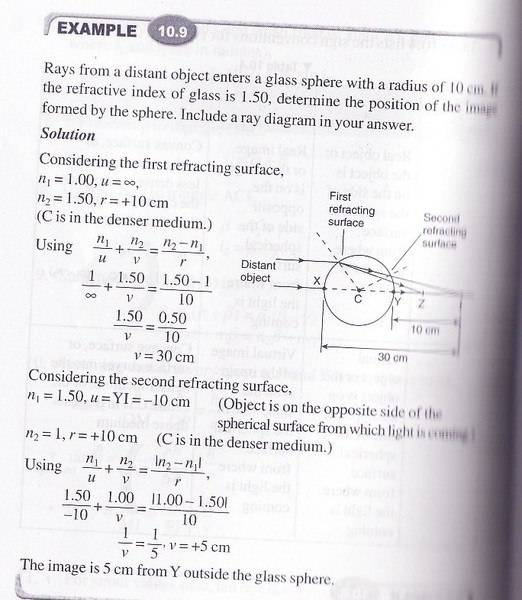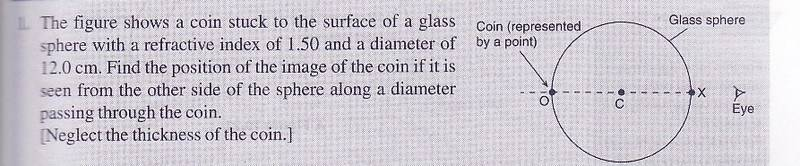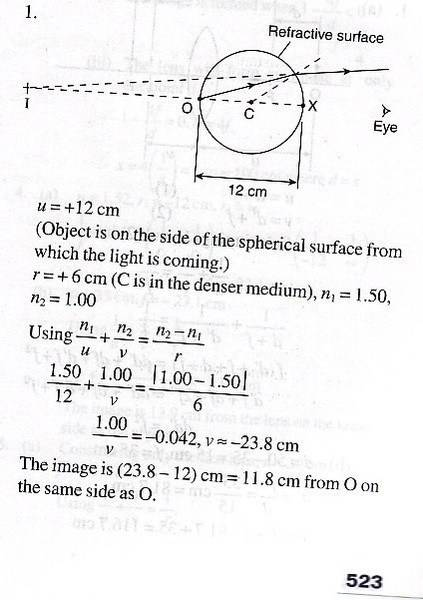# Refraction at a spherical surface

## Homework Statement## The Attempt at a Solution

I was kind of confuse with the use of refractive index.

For the first part of question, n1 = 1.00 and n2 = 1.50. From what I know, the object is at vacuum so the n1 = 1.00.

But why for the second part of the question, n1 = 1.50 and n2 = 1.00 ? After the first refracting surface, the image should be at the vacuum too. But why we use n1 = 1.50?

## Answers and Replies

tiny-tim
Science Advisor
Homework Helper
Welcome to PF!

Hi stpmmaths! Welcome to PF!I was kind of confuse with the use of refractive index.

For the first part of question, n1 = 1.00 and n2 = 1.50. From what I know, the object is at vacuum so the n1 = 1.00.

But why for the second part of the question, n1 = 1.50 and n2 = 1.00 ? After the first refracting surface, the image should be at the vacuum too. But why we use n1 = 1.50?

(I can't see your attachment yet, but …)

I assume that the light starts by going into the sphere from the air (or vacuum), so it's going from n = 1 to n = 1.5;

then it travels through the sphere (n = 1.5) until it hits the opposite surface and comes out again, so it's going from n = 1.5 to n = 1.Hi stpmmaths! Welcome to PF!(I can't see your attachment yet, but …)

I assume that the light starts by going into the sphere from the air (or vacuum), so it's going from n = 1 to n = 1.5;

then it travels through the sphere (n = 1.5) until it hits the opposite surface and comes out again, so it's going from n = 1.5 to n = 1.Ok..I understand a bit.

Now I came across with another question similar to this question(quite similar)

Quenstion:Answer:Why the n1 is 1.50 if we say the light starts from vacuum?#### Attachments

tiny-tim
Science Advisor
Homework Helper
Why the n1 is 1.50 if we say the light starts from vacuum?Because the light starts from the coin, inside the sphere, not from vacuum.Redbelly98
Staff Emeritus
Science Advisor
Homework Helper
Why the n1 is 1.50 if we say the light starts from vacuum?Look carefully at the arrows in the figure. Where do they start from?

EDIT: to clarify, look at the interface where the two rays meet. The ray arrows indicate they go from the glass into the air.

p.s. Hello tiny-tim!

Oo, I got it.
Thanks everyone.